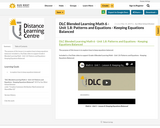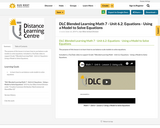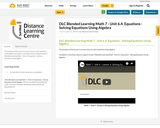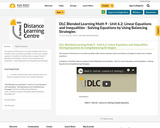Updating search results...

# 4 Results

View
Selected filters:
• balanced-equationsConditional Remix & Share Permitted
CC BY-NC-SA
Rating
0.0 stars

The purpose of this lesson is to explore how to keep equations balanced.

Included is a YouTube video to support Grade 6 Blended Learning Math - Unit 1.8: Patterns and Equations - Keeping Equations Balanced.

Subject:
Math
Material Type:
Activity/Lab
Homework/Assignment
Lesson
Provider:
Sun West Distance Learning Centre (DLC)
05/14/2019Conditional Remix & Share Permitted
CC BY-NC-SA
Rating
0.0 stars

The purpose of this lesson is to learn how to use balance scale models to solve equations.

Included is a YouTube video to support Grade 7 Blended Learning Math - Unit 6.2: Equations - Using a Model to Solve Equations.

Subject:
Math
Material Type:
Activity/Lab
Homework/Assignment
Lesson
Provider:
Sun West Distance Learning Centre (DLC)
06/12/2019Conditional Remix & Share Permitted
CC BY-NC-SA
Rating
0.0 stars

The purpose of this lesson is to learn how to solve equations using algebra.

Included is a YouTube video to support Grade 7 Blended Learning Math - Unit 6.4: Equations - Solving Equations Using Algebra.

Subject:
Math
Material Type:
Activity/Lab
Homework/Assignment
Lesson
Provider:
Sun West Distance Learning Centre (DLC)
06/12/2019Conditional Remix & Share Permitted
CC BY-NC-SA
Rating
0.0 stars

The purpose of this lesson is to model a problem with a linear equation and to use balance strategies to solve more complex equations.

Included is a YouTube video to support Grade 9 Blended Learning Math - Unit 6.2: Linear Equations and Inequalities - Solving Equations by Using Balancing Strategies.

Subject:
Math
Material Type:
Activity/Lab
Homework/Assignment
Lesson
Provider:
Sun West Distance Learning Centre (DLC)